# Real Numbers Exercise – 1.4

1. Without actually performing the long division, state whether the following rational numbers will have a terminating decimal expansion or a non-terminating repeating decimal expansions.

(i)13/3125

Solution:

3125 = 5×5×5×5×5 = 55

We know that in a rational number p/q, q is written as a factor of 2m×5n (where m and n are non-negative integers) then its decimal expansion is terminating.

The prime factor of 3125 = 55

So this factor can be written as 2m×5n (20×55) so the decimal expansion will be terminating.

(ii)17/8

Solution:

8 = 2×2×2 = 23

We know that in a rational number p/q, q is written as a factor of 2m×5n (where m and n are non-negative integers) then its decimal expansion is terminating.

The prime factor of 8 = 23

So this factor can be written as 2m×5n (23×50) so the decimal expansion will be terminating.

(iii)64/455

Solution:

455 = 4×7×13

We know that in a rational number p/q, q is written as a factor of 2m×5n (where m and n are non-negative integers) then its decimal expansion is terminating.

The prime factor of 455 = 4×7×13

So this factor cannot be written as 2m×5n so the decimal expansion will be of non-terminating repeating.

(iv)15/1600

Solution:

1600 = 2×2×2×2×2×5×5 = 26×52

We know that in a rational number p/q, q is written as a factor of 2m×5n (where m and n are non-negative integers) then its decimal expansion is terminating.

The prime factor of 1600 = 26×52

So this factor can be written as 2m×5n (26×52) so the decimal expansion will be terminating.

(v)29/343

Solution:

343 = 7×7×7

We know that in a rational number p/q, q is written as a factor of 2m×5n (where m and n are non-negative integers) then its decimal expansion is terminating.

The prime factor of 343 = 73

So this factor cannot be written as 2m×5n so the decimal expansion will be of non-terminating repeating.

Solution:

Denominator = 23 ×52

We know that in a rational number p/q, q is written as a factor of 2m×5n (where m and n are non-negative integers) then its decimal expansion is terminating.

The prime factor of denominator= 23 ×52

So this factor can be written as 2m×5n (23 ×52) so the decimal expansion will be terminating.

Solution:

Denominator = 22×57×75

We know that in a rational number p/q, q is written as a factor of 2m×5n (where m and n are non-negative integers) then its decimal expansion is terminating.

The prime factor of denominator = 22×57×75

So this factor cannot be written as 2m×5n so the decimal expansion will be of non-terminating repeating.

(viii)6/15

Solution: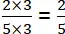Denominator = 5

We know that in a rational number p/q, q is written as a factor of 2m×5n (where m and n are non-negative integers) then its decimal expansion is terminating.

The prime factor of denominator = 5

So this factor can be written as 2m×5n (20 ×51) so the decimal expansion will be terminating.

(ix)35/50

Solution: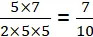Denominator = 10 = 2×5

We know that in a rational number p/q, q is written as a factor of 2m×5n (where m and n are non-negative integers) then its decimal expansion is terminating.

The prime factor of denominator = 2×5

So this factor can be written as 2m×5n (21 ×51) so the decimal expansion will be terminating.

(x)77/210

Solution:

Denominator = 2×3×5

We know that in a rational number p/q, q is written as a factor of 2m×5n (where m and n are non-negative integers) then its decimal expansion is terminating.

The prime factor of denominator = 2×3×5

So this factor cannot be written as 2m×5n so the decimal expansion will be of non-terminating repeating.

2. Write down the decimal expansions of those rational numbers in Question 1 above which have terminating decimal expansions.

(i)13/3125

Solution:

= 0.00416

(ii)17/8

Solution: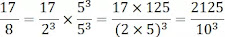= 2.125

(iii)64/455

Solution:

Decimal expansion is non-termination repeating.

(iv)15/1600

Solution:

= 0.009375

(v)29/343

Solution:

Decimal expansion is non-termination repeating.

Solution: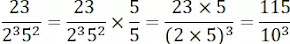= 0.115

Solution:

Decimal expansion is non-termination repeating.

(viii)6/15

Solution:

= 0.4

(ix)35/50

Solution: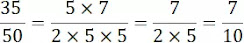= 0.7

(x)77/210

Solution:

Decimal expansion is non-termination repeating.

3. The following real numbers have decimal expansions as given below. In each case, decide whether they are rational or not. If they are rational and of the form P/q, what can you say about the prime factor of q?

(i) 43.123456789

Solution:

The decimal expansion is terminating so it is a rational number and it is of the form p/q. The prime factorization of q is of the form 2m×5n

(ii) 0.120120012000120000…

Solution:

The decimal expansion is non-terminating and non-recurring so it is an irrational number.

Solution:

The decimal expansion is non-terminating and repeating, so it is a rational number and it is of the form p/q. The prime factorization of q is any prime number other than 2m×5n